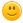# How to round numbers?

179 views
asked Oct 19, 2013 in Math
What is the rule for rounding a number. What do you do if it ends in .5, like 1.5?

answered Jul 21, 2014 by (424,180 points)
if over 5 go up

but if lower than 5 go down.
+1 vote
answered May 22, 2014 by (32,490 points)
The rule for .5, or 5 is to round up, if it is 4 or under round down if it is 5 or over round up!
+1 vote
answered Nov 25, 2013 by (32,100 points)
edited Apr 2, 2017I think that for rounding numbers, you round up if the number is half way in between.

For example, 1.5 is in between 1 and 2. Scine it is the middle number, you round up.

Round up if it is 5 and up.

Round down if it is 4 and below.

I agree with Wizkid.+1 vote
answered Oct 21, 2013 by (65,700 points)

Here are the basics.

1.) Look at the last digit in the number. Example: 23.421 (1 is last digit). If the number is 0, 1, 2, 3, or 4, you don't do anything and just drop  that digit. Example becomes 23.42

2.)  The next rule is more complicated and there is an easy way and more difficult, but accurate method. I'll start with the easy way.

Simple Way:

If the last digit ends in 5, 6, 7, 8 or 9, just round up to the next highest number by adding 1 to the number before the one you are dropping, 23.655 becomes 23.66, or 46.99 becomes 47.0, 65.6 becomes 65.7

More accurate way:

When the first digit dropped is 5 and there are no digits following or the digits following are zeros, make the preceding digit even.

Examples:

42.415  rounds to  42.42  (1 becomes even number)

42.485 rounds to 42.48  (no change since 8 is already even)

In most cases, the simple way is good enough, but to be more accurate use the odd/even rules with things ending in 5.